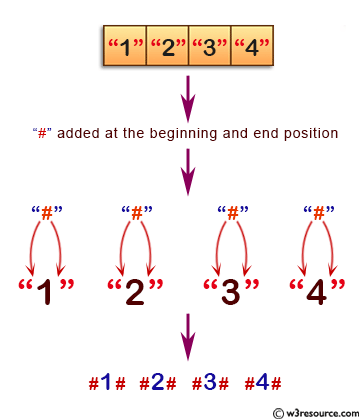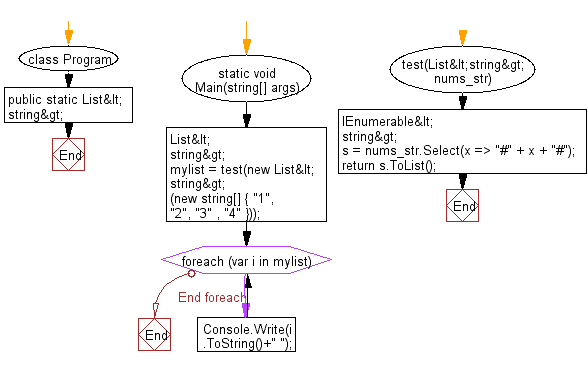﻿ C# Sharp exercises: Create a new list from a given list of strings where each element has '#' added at the beginning and end position - w3resource# C# Sharp Basic Algorithm Exercises: Create a new list from a given list of strings where each element has "#" added at the beginning and end position

## C# Sharp Basic Algorithm: Exercise-143 with Solution

Write a C# Sharp program to create a new list from a given list of strings where each element has "#" added at the beginning and end position.

Pictorial Presentation:Sample Solution:

C# Sharp Code:

``````using System;
using System.Collections.Generic;
using System.Linq;

namespace exercises
{
class Program
{
static void Main(string[] args)
{
List<string> mylist = test(new List<string>(new string[] { "1", "2", "3" , "4" }));
foreach(var i in mylist)
{
Console.Write(i.ToString()+" ");
}
}
public static List<string> test(List<string> nums_str)
{
IEnumerable<string> s = nums_str.Select(x => "#" + x + "#");
return s.ToList();
}
}
}
```
```

Sample Output:

`#1# #2# #3# #4#`

Flowchart:C# Sharp Code Editor:

Improve this sample solution and post your code through Disqus

What is the difficulty level of this exercise?

﻿